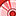# QlikView App Dev

Discussion Board for collaboration related to QlikView App Development.

Announcements
Join this live chat April 6, 10AM EST - QlikView to Qlik Sense REGISTER
cancel
Showing results for
Search instead for
Did you mean:Contributor

## Set analysis: Year to previous monthend calculation

Hi All,

I am struggling to calculate year to previous monthend sales in set analysis expression.

Example, If i select a date, it should calculate from year start to previous monthend sum of sales.

if i select any date in the month of Jan, it should calculate from 1st of Jan to 31st of Jan in that selected date.

This is the expression i am working on:

Sum({<year=, Quarter=, Month=, Week=, [End Date]=, End_Dt_Number={">=\$(=Num(YearStart(Max(End_Dt_Number))))<=\$(=Num(Monthend(AddMonths(Max(End_Dt_Number),-1))))"}>} Value)

Thanks in advance.

Labels (2)

• ### Set Analisys

1 Solution

Accepted SolutionsAnonymous
Not applicable

your expression looks good.
to solve your january problem I would define a variable
vNoOfMonth = if (num(month(End_Dt_Number)=1,0,-1)  // hold 0 in january and -1 in other months
then use this variable in your expression
<=\$(=Num(Monthend(AddMonths(Max(End_Dt_Number),vNoOfMonth))))

3 RepliesSpecialist II

I believe you are saying one thing and calculating another.  If you pick any day in Jan,

End_Dt_Number >= 01-Jan-2019 and End_Dt_Number <= 31-Dec-2018Anonymous
Not applicable

your expression looks good.
to solve your january problem I would define a variable
vNoOfMonth = if (num(month(End_Dt_Number)=1,0,-1)  // hold 0 in january and -1 in other months
then use this variable in your expression
<=\$(=Num(Monthend(AddMonths(Max(End_Dt_Number),vNoOfMonth))))Contributor
Author

Thanks... Working fine...